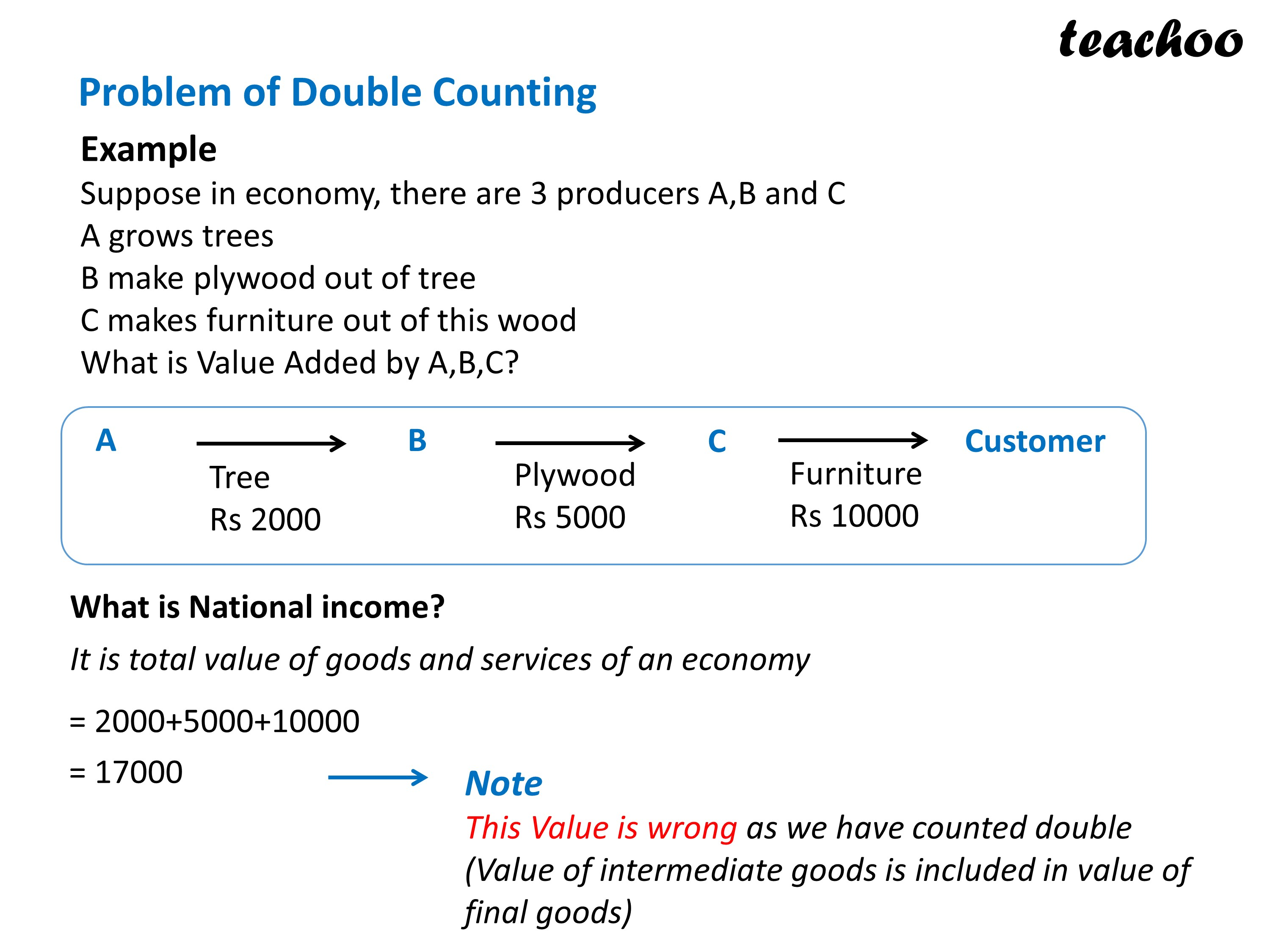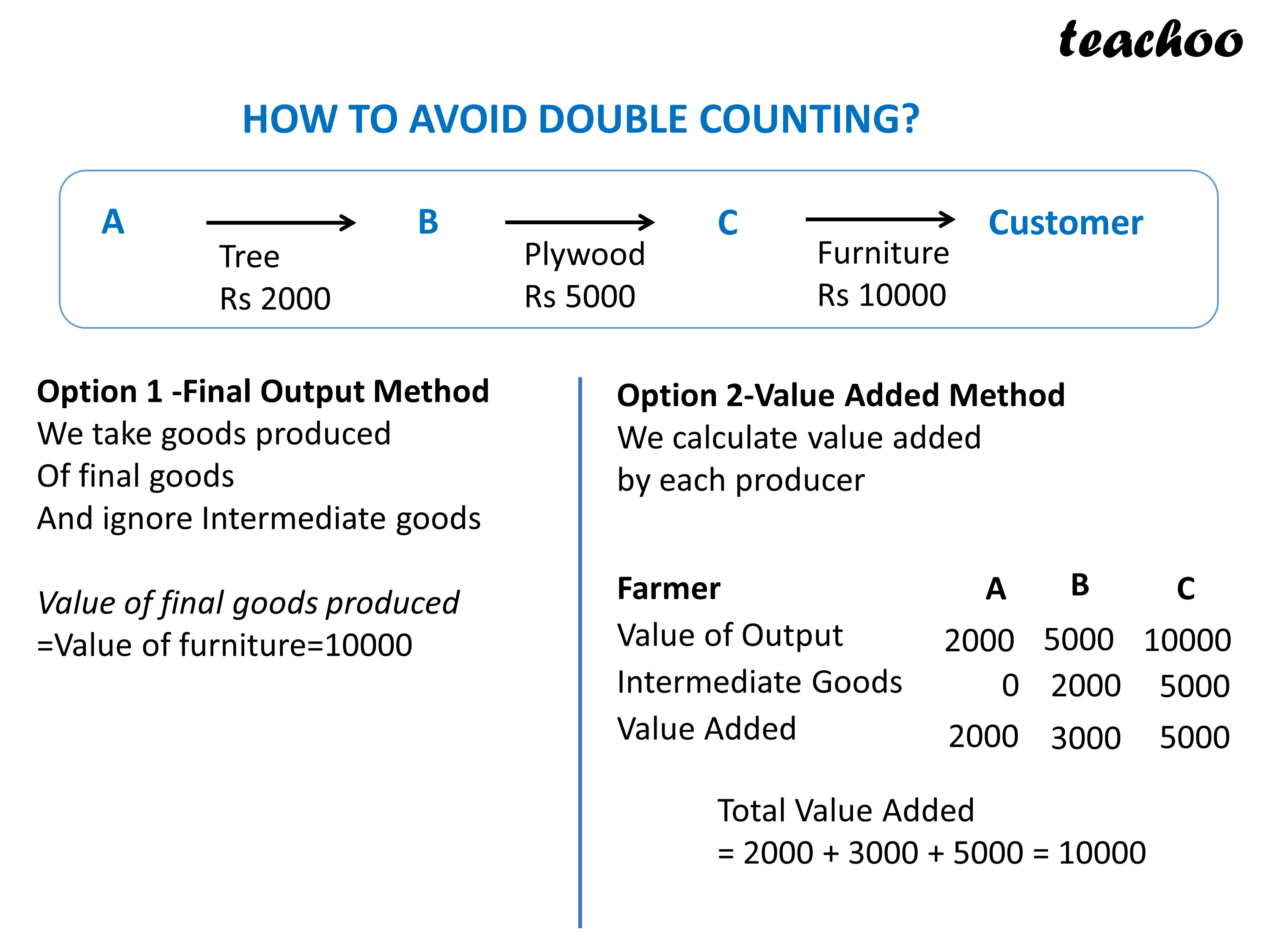Chapter 2 National Income - Part 3 Value Added Method

Economics Class 12
Macroeconomics## NCERT Questions

No questions in this part

## Question 1

What is the problem of Double Counting?

## Question 2

How to avoid problem of Double counting?

Learn in your speed, with individual attention - Teachoo Maths 1-on-1 Class

### Transcript

Problem of Double Counting in Value Added Method PROBLEM OF DOUBLE COUNTING Example Suppose in economy,there are 3 person A-B and C A grows trees B make plywood out of tree C makes furniture out of this wookd What is Value Added by A,B,C? A --> B -------- > C -------- > Customer Tree Plywood Furniture Rs 2000 Rs 5000 Rs 10000 What is National income? It is total value of goods and services of an economy =2000+5000+10000 =17000 ---> This Value is wrong as we have counted double (Value of intermediate goods is included in value of final goods) HOW TO AVOID DOUBLE COUNTING? Example Suppose in economy, there are 3 person A-B and C A grows trees B make plywood out of tree C makes furniture out of this wood What is Value Added by A,B,C? A --> B -------- > C -------- > Customer Tree Plywood Furniture Rs 2000 Rs 5000 Rs 10000 Option 1 -Final Output Method Value of final goods produced =Value of furniture=10000 Option 2-Value Added Method We calcualte value added by each producer A B C Farmer Value of Output 2000 5000 10000 Intermdiate Goods 0 2000 5000 Value Added 2000 3000 5000 total Value Added = 2000 + 3000 + 5000 = 10000 NCERT Questions No questions in this part Other Books Q1 What is the problem of Double Counting? Ans The problem of double counting arises when value of intermediate goods is also included in the value of final goods. Q2 How to avoid problem of Double counting? Ans There are 2 ways to avoid this problem: 1 Final Output Method In this method the value of final goods is added to determine the national income 2 Value Added Method In this method, sum total of value added by each producing unit should be taken in the national income.